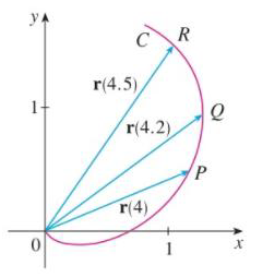Chapter 13.2, Problem 1E

Chapter
Section
Textbook Problem

# The figure shows a curve C given by a vector function r(t).(a) Draw the vectors r(4.5) − r(4) and r(4.2) − r(4).(b) Draw the vectors r ( 4.5 ) − r (4) 0.5 and r ( 4.2 ) − r (4) 0.2 (c) Write expressions for r'(4) and the unit tangent vector T(4).(d) Draw the vector T(4).(a)

To determine

To draw: The vectors r(4.5)r(4) and r(4.2)r(4).

Explanation

The vector r(4.5)r(4) is the vector joined from the points P to R in the curve C. The vector r(4.2)r(4) is the vector joined from the points P to Q in the curve C.

Draw the vectors r(4.5)r(4) and r(4

(b)

To determine

To draw: The vectors r(4.5)r(4)0.5 and r(4.2)r(4)0.2.

(c)

To determine

To write: The expression for the vector r(4) and the unit tangent vector T(4).

(d)

To determine

To draw: The vector T(4).

### Still sussing out bartleby?

Check out a sample textbook solution.

See a sample solution

#### The Solution to Your Study Problems

Bartleby provides explanations to thousands of textbook problems written by our experts, many with advanced degrees!

Get Started

#### In Exercises 5-8, find the limit. limx0(x+x)2x2x

Calculus: An Applied Approach (MindTap Course List)

#### 61/2

Applied Calculus for the Managerial, Life, and Social Sciences: A Brief Approach

#### True or False: If a number p is in the interval of convergence of then so is the number .

Study Guide for Stewart's Single Variable Calculus: Early Transcendentals, 8th